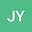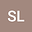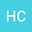Existence and multiple solutions for the critical fractional $p$-Kirchhoff type problems involving sign-changing weight functions
•••Senli Liu
Central South University School of Mathematics and Statistics
Author ProfileHaibo Chen
Department of Mathematics, Central South University
Author Profile## Abstract

The aim of this paper is to study the existence and multiplicity of nonnegative solutions for the following critical Kirchhoff equation involving the fractional $$p$$-Laplace operator $$(-\Delta)_{p}^{s}$$. More precisely, we consider

\begin{equation} \begin{cases}M\left(\iint_{\mathbb{R}^{2N}}\frac{|u(x)-u(y)|^{p}}{|x-y|^{N+ps}}dxdy\right)(-\Delta)_{p}^{s}u=\lambda f(x)|u|^{q-2}u+K(x)|u|^{p_{s}^{*}-2}u,&{\rm in}\ \Omega,\\ u=0,&{\rm in}\ \mathbb{R}^{N}\setminus\Omega,\\ \end{cases}\nonumber \\ \end{equation}

where $$\Omega\subset\mathbb{R}^{N}$$ is an open bounded domain with Lipschitz boundary $$\partial\Omega$$, $$M(t)=a+bt^{m-1}$$ with $$m>1,a>0,b>0$$, dimension $$N>sp$$, $$p_{s}^{*}=\frac{Np}{N-ps}$$ is the fractional critical Sobolev exponent, and the parameters $$\lambda>0,0<s<1<q<p<\infty$$. Applying Nehari manifold, fibering maps and Krasnoselskii genus theory, we investigate the existence and multiplicity of nonnegative solutions.

#### Peer review status:UNDER REVIEW

01 Mar 2020Submitted to Mathematical Methods in the Applied Sciences
07 Mar 2020Assigned to Editor
07 Mar 2020Submission Checks Completed
10 Jul 2020Reviewer(s) Assigned